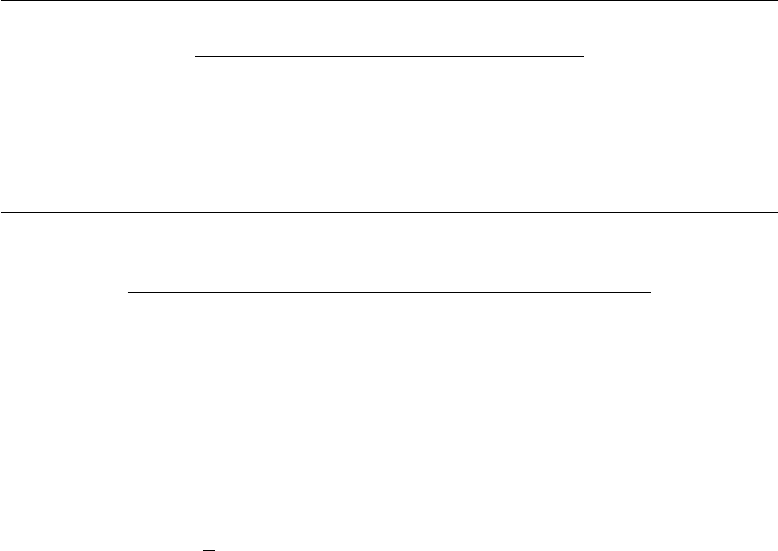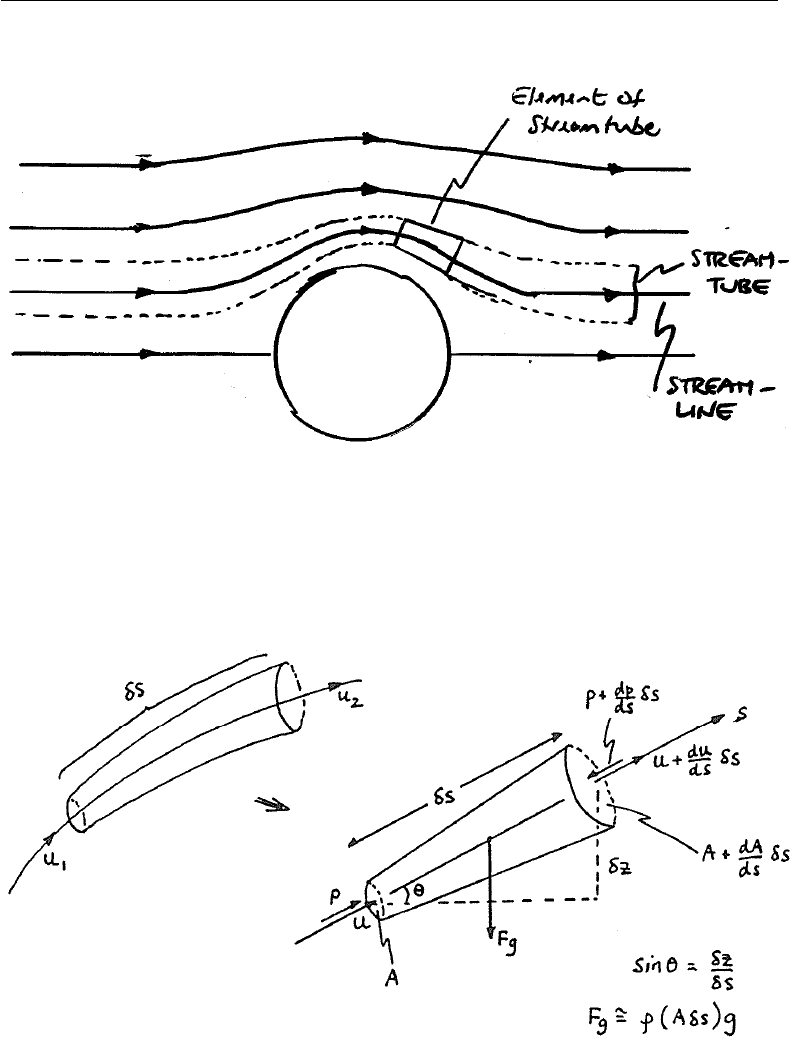# ENGR2000 Lecture Notes - Lecture 4: A Aa, Dynamic Pressure, Static Pressure

36 views16 pages
School
Department
Course
Professor2nd-Year Fluid Mechanics, Faculty of Science & Engineering, Curtin University
ENGR2000: FLUID MECHANICS
For Second-year Chemical, Petroleum, Civil & Mechanical Engineering
FLUID MECHANICS LECTURE NOTES
CHAPTER 4: THE BERNOULLI EQUATION
4.1 Introduction
We have already noted that the pressure in a ﬂuid is linked to its motion; it is
also linked to the hydrostatic pressure (consider a ﬂuid at rest). Bernoulli’s
equation, written below, deﬁnes the link:
p+1
2ρU2+ρgz =const. along a streamline (4.1)
where pis the actual pressure at a location in the ﬂow ﬁeld, U=|v|is the
speed of the ﬂuid (in the direction of the streamline) at that location and z
is the ‘up’ coordinate (from some horizontal datum) of that location. This
is an especially useful equation although there are limitations as to its use;
these will be discussed after (as a consequence of) its derivation.
4.2 Derivation of the Bernoulli equation
We will use the mass conservation and momentum equations. So, we need a
suitable control volume. Consider a stream-tube shown in Fig. X4.1 – it is
thin (so that the ﬂow velocity is constant across a given cross-section) tube
surrounding a streamline in the ﬂow. Note that we can think of the ﬂuid ﬂow
as comprising ‘bundles’ of stream-tubes. Where the ﬂow is fast (streamlines
close together), the stream-tube is thin; conversely, where the ﬂow slows
down, the sam stream-tube becomes thicker (mass-ﬂow conservation).
Chapter 4 Page 1
find more resources at oneclass.com
find more resources at oneclass.com
Unlock document

This preview shows pages 1-3 of the document.
Unlock all 16 pages and 3 million more documents.2nd-Year Fluid Mechanics, Faculty of Science & Engineering, Curtin University
FIGURE X4.1: Deﬁnition of a stream-tube (in ﬂow past a cylinder).
In Fig. 4.1a a stream-tube with (inﬁnitesimal) length δs is shown.
FIGURE 4.1: Stream-tube and inﬁnitesimal CV cut from it.
The same stream-tube is shown schematically in Fig. 4.1b and will be our
control volume. Since δs can be made arbitrarily short, we may consider the
s-axis to be a straight line; the application of the momentum equation will
Chapter 4 Page 2
find more resources at oneclass.com
find more resources at oneclass.com
Unlock document

This preview shows pages 1-3 of the document.
Unlock all 16 pages and 3 million more documents.2nd-Year Fluid Mechanics, Faculty of Science & Engineering, Curtin University
be along this axis so that we do not have to deal with vector quantities, i.e.
we eﬀectively have a one-dimensional problem.
First, note that if the value of a quantity, eg. D, is known at point s, then we
can write an expression for its value at s+δs by using a Taylor expansion,
viz.:
D(s+δs) = D(s) + δs
1
dD(s)
ds +δs2
2
d2D(s)
ds2+δs3
6
d3D(s)
ds3+.....
Since we can make δs arbitrarily small, (so δs >> δs2>> δs3....), we trun-
cate the above expansion to give a linear approximation:
D(s+δs)D(s) + dD(s)
ds δs (4.2)
or upon re-arrangement:
dD(s)
ds D(s+δs)D(s)
δs
where the approximation becomes exact in the limit, δs 0. (This is, of
course, the deﬁnition of diﬀerentiation of D, here with respect to s.) We will
use the form of Eqn. 4.2 repeatedly in the following.
We now apply mass conservation and the momentum equation:
(i) Mass Continuity:
Since the ﬂow is parallel to the streamline, ﬂow enters and leaves only through
the end faces of the CV. Using ‘mass ﬂow-rate in = mass ﬂow-rate out’,
having assumed steady and incompressible ﬂow:
ρUA =ρU+dU
ds δsA+dA
ds δs(= ˙m) (4.3)
(ii) Momentum Equation
We use the (scalar) momentum equation in the s-direction. Recall that:
Sum of applied forces = Rate of increase of momentum inside CV
- (momentum ﬂux INTO CV - momentum ﬂux OUT of CV)
(3.7b)
Chapter 4 Page 3
find more resources at oneclass.com
find more resources at oneclass.com
Unlock document

This preview shows pages 1-3 of the document.
Unlock all 16 pages and 3 million more documents.